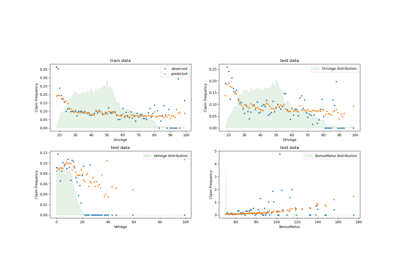# sklearn.metrics.mean_tweedie_deviance¶

sklearn.metrics.mean_tweedie_deviance(y_true, y_pred, *, sample_weight=None, power=0)[source]

Mean Tweedie deviance regression loss.

Read more in the User Guide.

Parameters
y_truearray-like of shape (n_samples,)

Ground truth (correct) target values.

y_predarray-like of shape (n_samples,)

Estimated target values.

sample_weightarray-like of shape (n_samples,), default=None

Sample weights.

powerfloat, default=0

Tweedie power parameter. Either power <= 0 or power >= 1.

The higher p the less weight is given to extreme deviations between true and predicted targets.

• power < 0: Extreme stable distribution. Requires: y_pred > 0.

• power = 0 : Normal distribution, output corresponds to mean_squared_error. y_true and y_pred can be any real numbers.

• power = 1 : Poisson distribution. Requires: y_true >= 0 and y_pred > 0.

• 1 < p < 2 : Compound Poisson distribution. Requires: y_true >= 0 and y_pred > 0.

• power = 2 : Gamma distribution. Requires: y_true > 0 and y_pred > 0.

• power = 3 : Inverse Gaussian distribution. Requires: y_true > 0 and y_pred > 0.

• otherwise : Positive stable distribution. Requires: y_true > 0 and y_pred > 0.

Returns
lossfloat

A non-negative floating point value (the best value is 0.0).

Examples

>>> from sklearn.metrics import mean_tweedie_deviance
>>> y_true = [2, 0, 1, 4]
>>> y_pred = [0.5, 0.5, 2., 2.]
>>> mean_tweedie_deviance(y_true, y_pred, power=1)
1.4260...


## Examples using sklearn.metrics.mean_tweedie_deviance¶